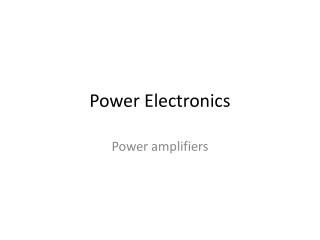DownloadDownload PresentationPower Electronics

# Power Electronics

Download Presentation## Power Electronics

- - - - - - - - - - - - - - - - - - - - - - - - - - - E N D - - - - - - - - - - - - - - - - - - - - - - - - - - -
##### Presentation Transcript

1. Power Electronics Power amplifiers

2. Figure 12.1-2. Thermodynamic context of power amplifier

3. Figure 12.1–2. Thermal resistances.

4. Figure 12.3–1 The pin junction: (a) Cross–section (b) Potentials and E–fields in reverse–bias (c) Injected carrier levels in forward bias

5. Figure 12.4–1 Class-A circuit using BJT drivers.

6. Figure 12.4–2. (a) Class-A circuit consisting of BJT driver and simple current mirror. (b) Waveforms for the class-A amplifier.

7. Figure 12.5–1. Class-B topology with complementary push-pull BJTs

8. Figure 12.5-2. For purposes of plot analysis we have let VS = 10V. By inspection it appears that VL (worst) is approximately at 6.4V. And this is confirmed by equation 12.5-5 (below).

9. Figure 12.7–1. Crossover distortion effects with class-B

10. Figure 12.7–3. Principles of the class-AB amplifier.

11. Figure 12.7–2. Class-AB diode bias of transistor pair

12. Figure 12.7–3. The Darlington pair IE2 = (2 +1) x IE1

13. IE2 = (2 +1) x (IE1 – IR1) IE2 = (2 +1) x (IC1 – IR2) IE2 = (2 +1) x IC1

14. Figure 12.9–1. Circuits configurations using stacked power opamps and input buffer

15. Figure 12.9–2. The power bridge: (a) the opamp pair (b) output transistor operation.

16. Figure 12.10–2a. PWM (pulse-width modulation) circuit for front end of class-D amplifier. Figure 12.10–2b. Use of sawtooth to accomplish PWM